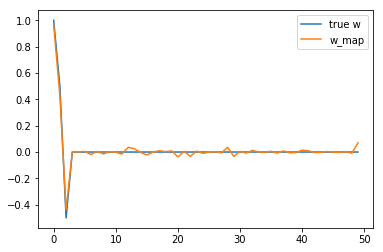# LASSO REGRESSION AND ITS IMPLEMENTATION WITH PYTHON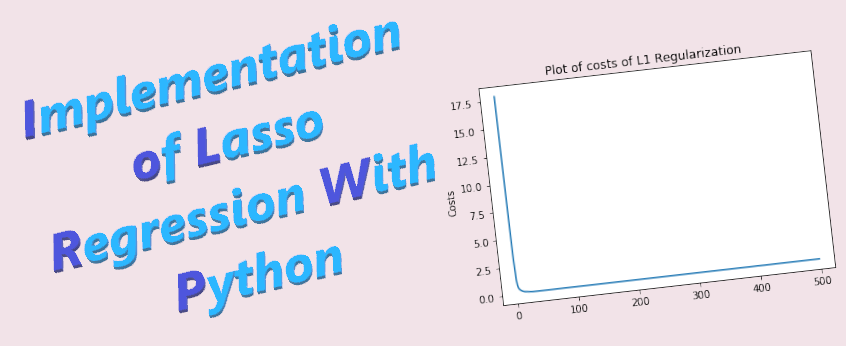Hi Everyone! Today, we will learn about Lasso regression/L1 regularization, the mathematics behind ridge regression and how to implement it using Python!

To build a great foundation on the basics, let’s understand few points given below:

• Firstly, let us have a look at the Sum of square of errors function, that is defined as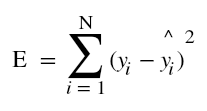• It is also important to note that the first requirement that should be fulfilled for any data set that we want to use for making machine learning models is that the data points should be random in nature and data size should be large.
• But this requirement is not fulfilled sometimes. That is, in some cases, number of features/dimensions(D) is greater than the number of samples/observations(N). Thus, the data set becomes fatty(D >> N) in nature instead of skinny(D << N).
• One thing to be noted is that even completely random noise can also improve R squared. But, this is very unwanted. We don’t want to let noise or unwanted features alter our outputs. This can be achieved by means of regularization.
• In case of L1 regularization, few weights, corresponding to the most important features, are kept non-zero and other/ most of them are kept equal to zero.
• For any data set which is random in nature, it should follow Gaussian distribution.
• Any Gaussian distribution is defined by its mean, µ and variance,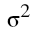and is represented bywhere X is the input matrix.
• For any point xi, the probability of xi is given by the expression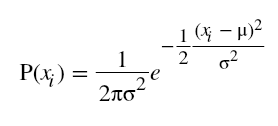.
• Also, because the occurrence of each xi is independent to the occurrence of other, the joint probability of each of them is given by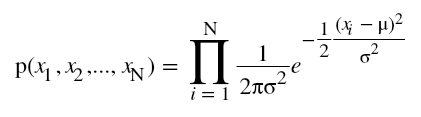• Also, linear regression is the solution which gives the maximum likelihood to the line of best fit.
• Now, the question arises, what is likelihood? Likelihood is defined as the probability of data X given a parameter of interest, in our case, it’s µ. So, likelihood function is defined as.
• Linear regression maximizes this function for the sake of finding the line of best fit. We do this by finding the value of µ for which this function is maximized and we can say that it is very likely that our data has come from a population that has µ as mean.
• For solving this, first we take the natural log of the likelihood function(L), then differentiate L wrt  µ and then equate this to zero.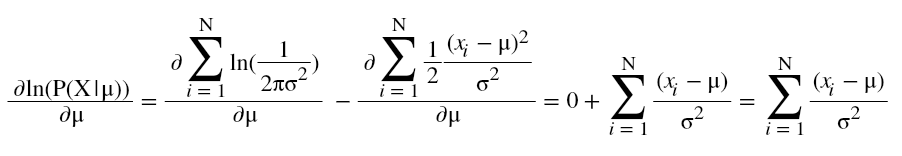Hence. at this value of µ, the likelihood function is maximized.

• One thing to be noted here is that maximizing likelihood function L is equivalent to minimizing error function E. Also, y is Gaussian distributed with mean transpose(w)*X and variance sigma-square ororwhere ε is Gaussian distributed noise with zero mean and sigma-square variance.
• This is equivalent to saying that in linear regression, errors are Gaussian and the trend is linear.
• Now, let’s understand why regularization was introduced. The answer is outliers! In the presence of outliers, the linear regression gets the line of best fit diverted from the real trend. This is because it follows the method of least squares and in order to minimize the error, it makes the trend line bent towards the outliers. This makes the prediction less accurate and far from what could be in the absence of outliers. To handle this problem, the method of Regularization was introduced.
• L1 regularization uses L1 norm as a penalty term.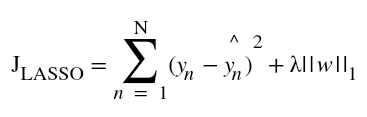• Plain squared error maximizes likelihood as shown above. But now, since we have two terms in the cost function, we no longer do this. We now have two probabilities, one is likelihood probability and other one is prior. Likelihood is given by:and prior is given by:• P(w) is called prior because it represents our prior beliefs about w. Thus, now, J is proportional to -ln(P(Y|X, w))-ln(P(w)). Also, by Baye’s rule, we get, P(w|Y,X) is proportional to P(Y|X,w)*P(w). P(w|Y,X) is called the Posterior probability. The method of maximizing P(w|Y,X) is called Maximizing A Posterior or MAP.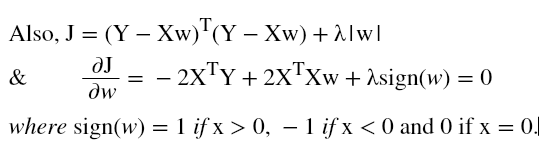Now, let’s see how to implement L1 regularization or Lasso Regression by using Gradient Descent(I will be covering gradient descent in a separate post).

In :
```from __future__ import print_function, division
from builtins import range

import numpy as np # importing numpy with alias np
import matplotlib.pyplot as plt # importing matplotlib.pyplot with alias plt
```
In :
```No_of_observations = 50
No_of_Dimensions = 50

X_input = (np.random.random((No_of_observations, No_of_Dimensions))-0.5)*10 #Generating 50x50 matrix forX with random values centered round 0.5
w_dash =  np.array([1, 0.5, -0.5] + *(No_of_Dimensions-3)) # Making first 3 features significant by setting w for them as non-zero and others zero
Y_output = X_input.dot(w_dash) + np.random.randn(No_of_observations)*0.5 #Setting Y = X.w + some random noise
```
In :
```costs = [] #Setting empty list for costs
w = np.random.randn(No_of_Dimensions)/np.sqrt(No_of_Dimensions) #Setting w to random values
L1_coeff = 5
learning_rate = 0.001 #Setting learning rate to small value so that the gradient descent algo doesn't skip the minima
```
In :
```for i in range(500):
Yhat = X_input.dot(w)
delta = Yhat - Y_output #the error between predicted output and actual output
w = w - learning_rate*(X_input.T.dot(delta) + L1_coeff*np.sign(w)) #performing gradient descent for w
meanSquareError = delta.dot(delta)/No_of_observations #Finding mean square error
costs.append(meanSquareError) #Appending mse for each iteration in costs list

```
In :
```plt.plot(costs)
plt.title("Plot of costs of L1 Regularization")
plt.ylabel("Costs")
plt.show()
```In :
```print("final w:", w) #The final w output. As you can see, first 3 w's are significant , the rest are very small
```
```final w: [  9.65816491e-01   4.27099719e-01  -4.39501114e-01   7.26803718e-04
1.44676529e-03   4.29653783e-03  -1.88827800e-02   5.01402266e-03
-1.45435498e-02   2.98832870e-03  -1.94071569e-03  -1.47917010e-02
3.56488642e-02   2.44495593e-02  -3.40885499e-03  -2.23948913e-02
-8.56983401e-04   1.00292301e-02   3.33973800e-03   8.51922055e-03
-3.72198952e-02   5.31823613e-03  -3.35052948e-02   7.15853488e-03
-1.00094617e-02  -1.44190084e-03   2.96771082e-03  -6.51081371e-03
3.54465569e-02  -3.30111666e-02   4.42377796e-03  -7.87768360e-03
1.26511065e-02  -5.43831611e-04  -4.58914064e-04   5.53972101e-03
-8.31677251e-03   8.63159114e-03  -6.17622135e-03  -3.08958154e-03
1.39908214e-02   9.34415972e-03  -3.76350383e-03  -2.16322570e-03
3.84337810e-03  -6.68382801e-04  -2.84473367e-03   2.48744388e-03
-8.91564845e-03   6.97568406e-02]
```
In :
```# plot our w vs true w
plt.plot(w_dash, label='true w')
plt.plot(w, label='w_map')
plt.legend()
plt.show()
```